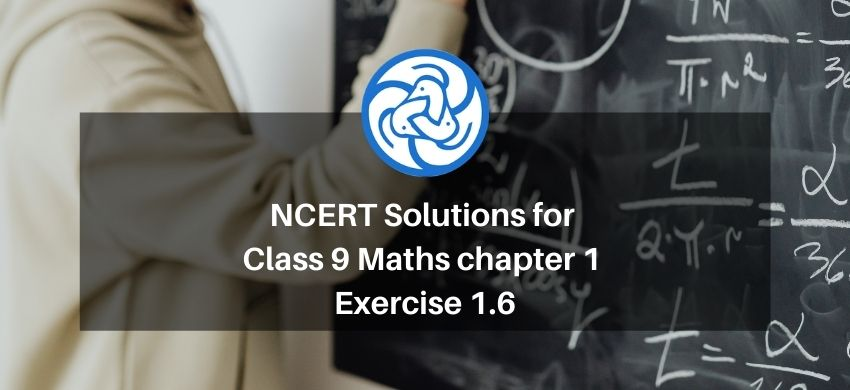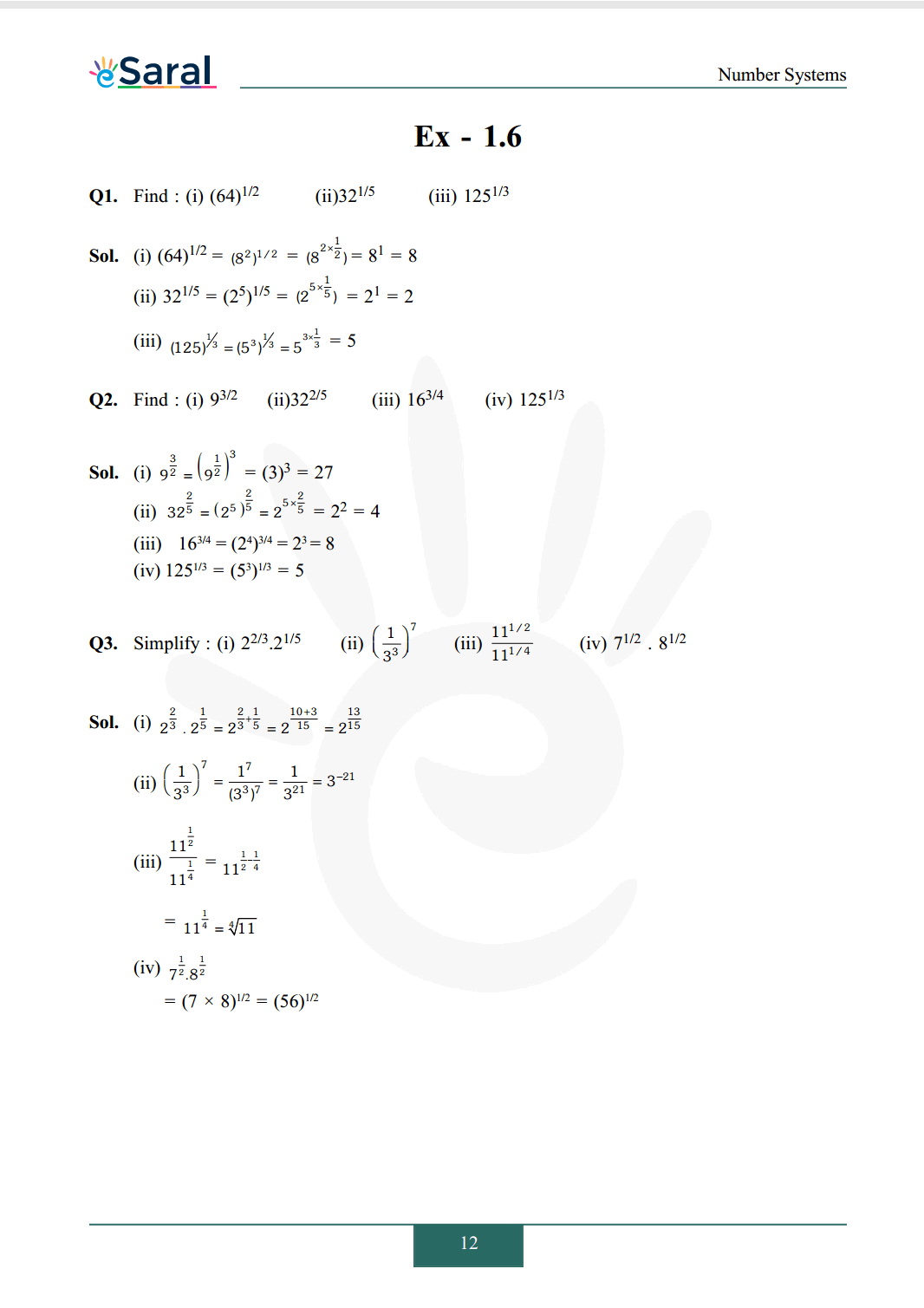Download the eSaral app and start learning from Kota's top IITians and doctors.

# NCERT Solutions for Class 9 Maths chapter 1 Exercise 1.6 - Number System - Free PDF DownloadHey, are you a class 9 Student and Looking for Ways to Download NCERT Solutions for Class 9 Maths chapter 1 Exercise 1.6? If Yes then you are at the right place.

Here we have listed Class 9 maths chapter 1 exercise 1.6 solutions  in PDF that is prepared by Kota’s top IITian’s Faculties by keeping Simplicity in mind.

If you want to score high in your class 9 Maths Exam then it is very important for you to have a good knowledge of all the important topics, so to learn and practice those topics you can use eSaral NCERT Solutions.

So, without wasting more time Let’s start.

####### All Questions of Chapter 1 Exercise 1.6

Once you complete the chapter 1 then you can revise Ex. 1.6 by solving following questions

Q1. Find :

(i) $(64)^{1 / 2}$

(ii) $32^{1 / 5}$

(iii) $125^{1 / 3}$

Q2. Find :

(i) $9^{3 / 2}$

(ii) $32^{2 / 5}$

(iii) $16^{3 / 4}$

(iv) $125^{1 / 3}$

Q3. Simplify :

(i) $2^{2 / 3} \cdot 2^{1 / 5}$

(ii) $\left(\frac{1}{3^{3}}\right)^{7}$

(iii) $\frac{11^{1 / 2}}{11^{1 / 4}}$

(iv) $7^{1 / 2} \cdot 8^{1 / 2}$

Watch Class 9 Maths Revision Quick Videos.